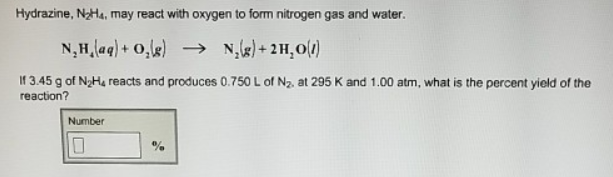# Problem: Hydrazine, N2H4, may react with oxygen to form nitrogen gas and water. N2H4 (aq) + O2 (g) → N2 (g) + 2 H2O (l) If 3.45 g of N2H4 reacts and produces 0.750 L of N2, at 295 K and 1.00 atm, what is the percent yield of the reaction?

###### FREE Expert Solution
100% (471 ratings)###### Problem Details

Hydrazine, N2H4, may react with oxygen to form nitrogen gas and water.

N2H4 (aq) + O(g) → N2 (g) + 2 H2O (l)

If 3.45 g of N2H4 reacts and produces 0.750 L of N2, at 295 K and 1.00 atm, what is the percent yield of the reaction?Frequently Asked Questions

What scientific concept do you need to know in order to solve this problem?

Our tutors have indicated that to solve this problem you will need to apply the Percent Yield concept. You can view video lessons to learn Percent Yield. Or if you need more Percent Yield practice, you can also practice Percent Yield practice problems.